Linear Elastic Material
For a linear elastic material, Hooke’s law relates the stress tensor to the elastic strain tensor:
where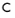is the 4th order elasticity tensor, “:” stands for the double-dot tensor product (or double contraction). The elastic strain εel is the difference between the total strain ε and all inelastic strains εinel. There may also be an extra stress contribution σex with contributions from initial stresses and viscoelastic stresses. In case of geometric nonlinearity, the second Piola-Kirchhoff stress tensor and the Green-Lagrange strain tensor are used.
The elastic strain energy density is
This expression assumes that the initial stress contribution is constant during the straining of the material.
Tensor vs. Matrix Formulations
Because of the symmetry, the strain tensor can be written as the following matrix:A similar representation applies to the stress tensor:Due to the symmetry, the elasticity tensor can be completely represented by a symmetric 6-by-6 matrix as:which is the elasticity matrix.
Isotropic Material and Elastic Moduli
In this case, the elasticity matrix becomesDescription Variable D(E,ν) D(E,G) D(K,G) D(λ,μ) Young’s modulus E = E E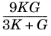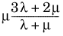Poisson’s ratio ν = ν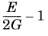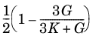Bulk modulus K =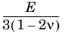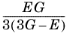K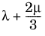Shear modulus G =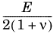G G μ λ =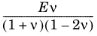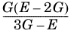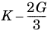λ μ =G G μ Pressure-wave speed cp =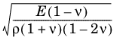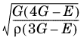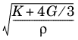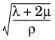Shear-wave speed cs =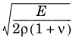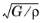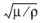According to Table 3-1, the elasticity matrix D for isotropic materials is written in terms of Lamé parameters λ and μ,or in terms of the bulk modulus K and shear modulus G:Orthotropic and Anisotropic Materials
There are two different ways to represent orthotropic or anisotropic data. The Standard (XX, YY, ZZ, XY, YZ, XZ) material data ordering converts the indices as:thus, Hooke’s law is presented in the form involving the elasticity matrix D and the following vectors:COMSOL Multiphysics uses the complete tensor representation internally to perform the coordinate system transformations correctly.
Beside the Standard (XX, YY, ZZ, XY, YZ, XZ) Material data ordering, the elasticity coefficients can be entered following the Voigt notation. In the Voigt (XX, YY, ZZ, YZ, XZ, XY) Material data ordering, the sorting of indices is:The last three rows and columns in the elasticity matrix D are thus swapped.
Orthotropic Material
The elasticity matrix for orthotropic material in the Standard (XX, YY, ZZ, XY, YZ, XZ) Material data ordering has the following structure:where the components are as follows: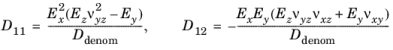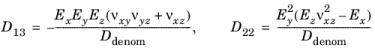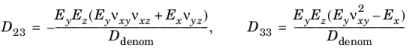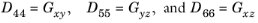where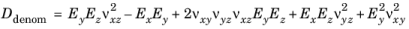The values of Ex, Ey, Ez, νxy, νyz, νxz, Gxy, Gyz, and Gxz are supplied in designated fields in the physics interface. COMSOL Multiphysics deduces the remaining components—νyx, νzx, and νzy—using the fact that the matrices D and D1 are symmetric. The compliance matrix has the following form: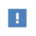The values of νxy and νyx are different for an orthotropic material. For a certain set of given material data, you must make sure that the definition of the indexes is consistent with the definition used in COMSOL Multiphysics.
The elasticity matrix in the Voigt (XX, YY, ZZ, YZ, XZ, XY) Material data ordering changes the sorting of the last three elements in the elasticity matrix: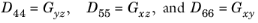Anisotropic Material
In the general case of fully anisotropic material, you provide explicitly all 21 components of the symmetric elasticity matrix D, in either Standard (XX, YY, ZZ, XY, YZ, XZ) or Voigt (XX, YY, ZZ, YZ, XZ, XY) Material data ordering.
Axial Symmetry
For the linear elastic material, the stress components in coordinate system are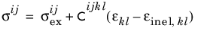The user input D matrix always contains the physical components of the elasticity tensor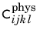and the corresponding tensor components are computed internally according to: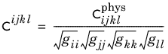For an isotropic material: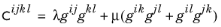where λ and μ are the first and second Lamé elastic parameters and g is the metric tensor.
For a hyperelastic material, the second Piola-Kirchhoff stress tensor is computed as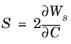which is computed as the contravariant components of the stress in the local coordinate system: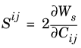The energy variation is computed as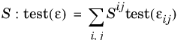which can be also written as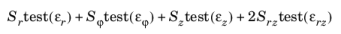Entropy and Thermoelasticity
The free energy for the linear thermoelastic material can be written as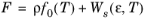where the strain energy density Ws(ε, T) is given by Equation 3-12. Hence, the stress can be found as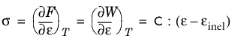and the entropy per unit volume can be calculated as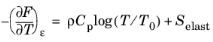where T0 is a reference temperature, the volumetric heat capacity ρCp can be assumed to be independent of the temperature (Dulong-Petit law), and the elastic entropy is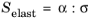where α is the thermal expansion coefficient tensor. For an isotropic material, it simplifies into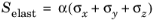The heat balance equation can be written as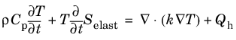where k is the thermal conductivity matrix, and the heat source caused by the dissipation is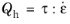where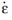is the strain-rate tensor and the tensor τ represents all possible inelastic stresses (for example, a viscous stress).
Using the tensor components, the heat balance can be rewritten as:
In many cases, the second term can be neglected in the left-hand side of Equation 3-13 because all Tαmn are small. The resulting approximation is often called uncoupled thermoelasticity.